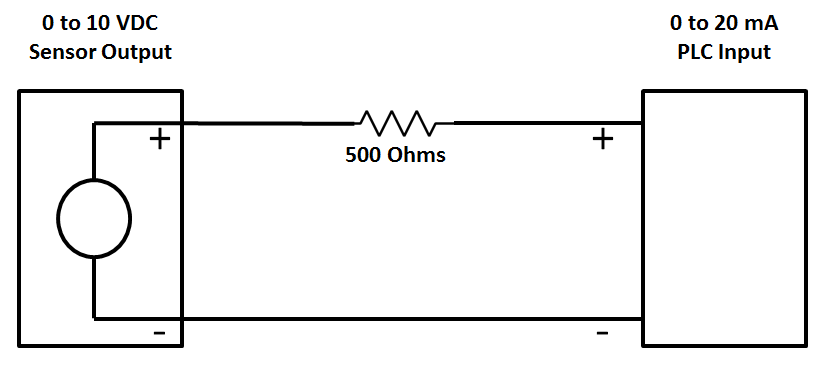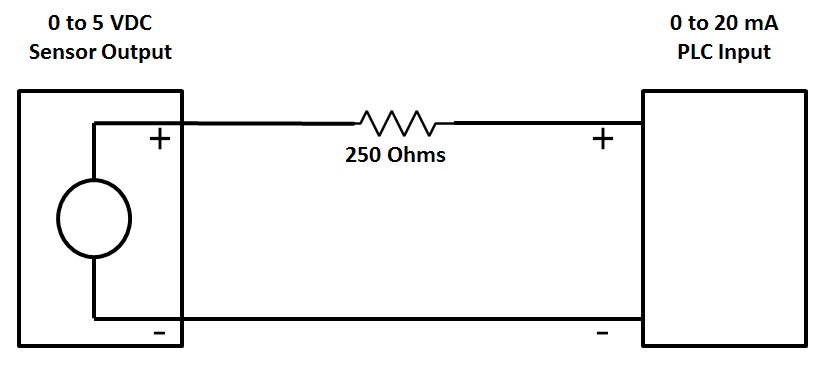### Convert 0-10 V to 0-20 mA Using Resistor

It is extremely simple to measure 0-10Vdc signal with a device that will measure only Current inputs. If Current input module available will accept a 0-20mA signal, but may not accept a 0-10Vdc signal directly.
Basically, Ohms law is used to calculate a resistor value in order to convert the 0-10Vdc signal to a current.
Voltage to Current Conversion Circuit Diagram

#### Example (0-10 VDC to 0-20 mA)

Ohms law states: R = V/I where V is the Voltage, I is the current and R is the resistance

R = 10V/0.020A = 500 Ohms0-10 VDC to 0-20 mA Conversion Circuit Diagram

I= V/R = 0/500   = 0mA

I= V/R = 10/500 = 0.02A = 20mA

#### Example (0-5 VDC to 0-20 mA)

Ohms law states: R = V/I where V is the Voltage, I is the current and R is the resistance

R = 5V/0.020A = 250 Ohms0-5 VDC to 0-20 mA Conversion Circuit Diagram

I= V/R = 0/250   = 0mA

I= V/R = 5/250 = 0.02A = 20mA

#### Note:-

To avoid damage you must ensure that the external current source has short-circuit protection in all conductor cases.
The external resistor is a source of error because of its dependency on temperature and its inaccuracy.
In order to obtain measuring results that are as precise as possible it is recommended to use resistors with tolerances that are as small as possible.

1.I want to learn

1.2.I enjoyed reading this blog post. It was inspiring and informative. Thank you. nata coaching centres in chennai

3.I enjoy this. Bit I will like to see a conversion from 0-10v to 4-20mA

4.Hello there, do you think this would be a good alternative to "monitor" battery levels?
we Run a 12V battery and our DAQ can read 0-20mA, so I could in theory that you showed here, run a 600Ohmns and get my current?
Thank you

1.Batteries are a little more complicated than that. A fully charged 12V battery could be closer to 13 or 14 volts. If it's a car battery and the engine is running, you may see 15V. So, it'd probably be safer to use a 15V/.02A= 750ohm resistor.

Also, if constantly drawing 20mA, you're battery may die pretty quick depending on how big it is. I'd invest in something designed to read voltage instead of current.

5.I wanted to thank you for this great read!! I definitely enjoying every little bit of it I have you bookmarked to check out new stuff you post. Article Rewriter Tool